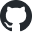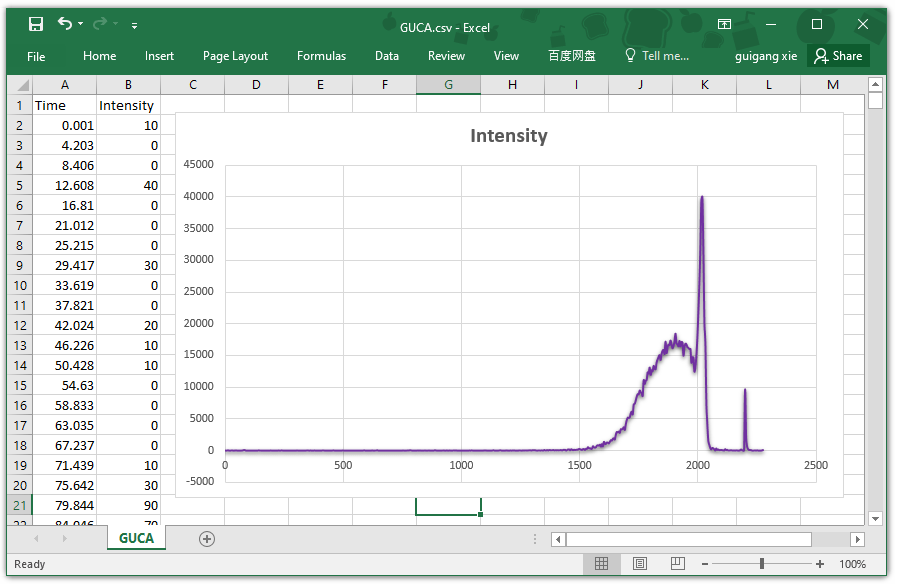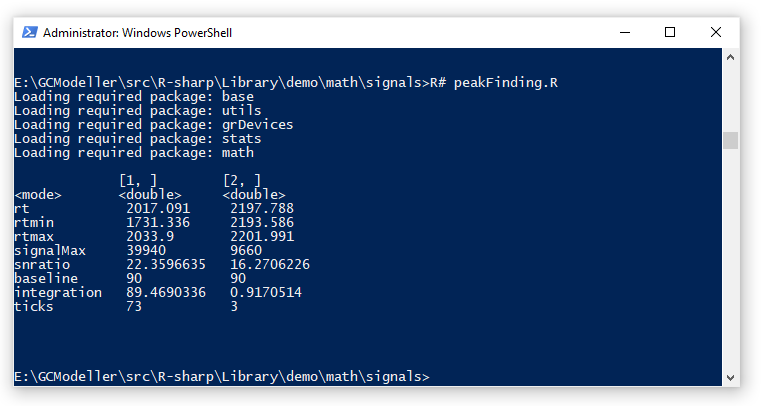####https://github.com/xieguigang/sciBASIC/tree/master/Data_science/Mathematica/SignalProcessing

• 对于靶向定量计算，我们只需要按照MRM的离子对得到对应的离子对目标的TIC进行峰识别；或者在GCMS靶向定量计算中，通过定量离子进行在SIM模式下取得对应的XIC进行峰识别
• 对于非靶向定量计算而言，虽然非靶向数据相较于靶向数据非常复杂。但是我们任然可以通过将一级质谱原始数据转换为XIC色谱图之后进行峰识别操作。

## 通过累加线进行峰识别

          /\
/  \
/\/    \
_____/        \______• 空信号区域的累加线的值基本无变化，无变化意味着这一段区间内的累加线基本与X坐标轴平行
• 当累加线到达峰的左边的时候，累加线会突然逐步增大。这个时候累加线的斜率是大于零的
• 当累加线到达峰的右边的时候，累加线又会重新趋向于与X坐标轴平行

## 累加线算法的VisualBasic代码实现

1. 首先，我们就需要从原始的信号数据之中计算出累加线数据
2. 然后，从累加线中计算出一个斜率数据
3. 之后，将斜率数据中大于阈值的区域都给拿出来
4. 将区域重新映射回原始信号数据中就得到所有的信号峰数据了。

<Extension>
Public Function AccumulateLine(signals As ITimeSignal(), baseline As Double) As Vector2D()
Dim accumulate#
Dim sumALL# = Aggregate t As ITimeSignal In signals
Let x As Double = t.intensity - baseline
Where x > 0
Into Sum(x)
Dim ay As Func(Of Double, Double) =
Function(into As Double) As Double
into -= baseline
accumulate += If(into < 0, 0, into)
Return (accumulate / sumALL) * 100
End Function
Dim accumulates As Vector2D() = signals _
.Select(Function(tick)
Return New Vector2D(tick.time, ay(tick.intensity))
End Function) _
.ToArray

Return accumulates
End Function

Private Iterator Function sin(line As Vector2D()) As IEnumerable(Of Vector2D)
Dim A As Vector2D
Dim B As Vector2D
Dim sinA, sinC As Double

For Each con As SlideWindow(Of Vector2D) In line.SlideWindows(winSize:=2, offset:=1)
If con.Length = 2 Then
A = con.First
B = con.Last
sinA = B.y - A.y
sinC = EuclideanDistance(New Double() {A.x, A.y}, New Double() {B.x, B.y})

'      B
'     /|
'    / |
'   /  |
' A ---- X

Yield New Vector2D(A.x, sinA / sinC)
End If
Next
End Function

Private Iterator Function filterBySinAngles(angles As Vector2D()) As IEnumerable(Of SeqValue(Of Vector2D()))
Dim buffer As New List(Of Vector2D)
Dim i As i32 = 0

For Each a As Vector2D In angles
If a.y <= sin_angle Then
If buffer > 0 Then
buffer += a

Yield New SeqValue(Of Vector2D()) With {
.i = ++i,
.value = buffer.PopAll
}
End If
Else
buffer += a
End If
Next

If buffer > 0 Then
Yield New SeqValue(Of Vector2D()) With {
.i = ++i,
.value = buffer.PopAll
}
End If
End Function

rtmin = region.value.First.x - dt
rtmax = region.value.Last.x + dt
area = line((time >= rtmin) & (time <= rtmax))

' 因为Y是累加曲线的值，所以可以近似的看作为峰面积积分值
' 在这里将区间的上限的积分值减去区间的下限的积分值即可得到当前的这个区间的积分值（近似于定积分）
Yield New SignalPeak With {
.integration = area.Last.y - area.First.y,
.region = rawSignals((time >= rtmin) & (time <= rtmax)),
.baseline = baseline
}

## R#脚本中使用峰识别

' imports "signalProcessing" from "signalKit";

<ExportAPI("findpeaks")>
Public Function FindAllSignalPeaks(signal As GeneralSignal,
Optional baseline As Double = 0.65,
Optional cutoff As Double = 3) As SignalPeak()

Return New ElevationAlgorithm(cutoff, baseline) _
.FindAllSignalPeaks(signal) _
.ToArray
End Functionimports "signalProcessing" from "signalKit";

\${dirname(@script)}/GUCA.csv
|> (function(signal) as.signal(signal[, "Time"], signal[, "Intensity"]))
|> findpeaks(
baseline = 0.7,
cutoff   = 8
)
|> as.data.frame
|> t
|> print
;Latest posts by 谢桂纲 (see all)

### Order by Date Name Attachments

#### One response

1. […] 对于将上面所展示的XIC谱图里面所出现的峰的保留时间段位置给解析出来的操作，就是峰查找的操作了。进行峰查找操作，我们可以基于信号累加算法来实现。 […]

来自中国
1.2.3.查询字符串以["起始，以"]结尾： SELECT * FROM biodeepdb_full.metabolite WHERE INSTR(name, '["') = 1 AND INSTR(name, '"]') = LENGTH(name) - LENGTH('"]') + 1 ORDER…

4.删除mysql表中重复的行数据  delete t1 from metabolite t1 where t1.id in (select t2.id from (select id, name`, formula, hashcode2, row_number() over(partition…

5.